TigerJython4Kids micro:bit Robotik
sensor
 Deutsch   English   Français

## 3. ACCELEROMETER### YOU LEARN HERE...

 how to use the accelerometer to detect changes in position and movements of the micro:bit.

### SENSOR VALUES

The acceleration sensor (also called accelerometer) measures the constant gravitational acceleration of approx 10 m/s2, which points vertically downwards and the accelerations caused by movements (changes of velocity).

 The acceleration sensor is clearly visible on the board. Similar sensors are also part of most smartphones. The sensor measures the acceleration (including gravitational acceleration) in the x, y, and z directions. From these components, the pitch and the roll angles may be calculated.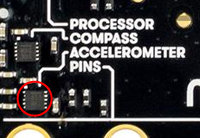It is similar to the values displayed in a aircraft (artificial horizon). If you tilt the board forwards or backwards, the pitch angle will change, while the sideways tilt will change the roll angle.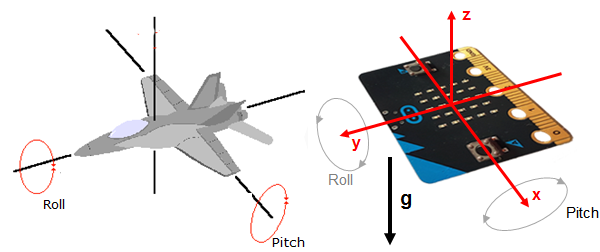In the program you use the accelerometer object and call accelerometer.get_x(), accelerometer.get_y() or accelerometer.get_z(). The sensor values are in the range of about -2000 to 2000 and correspond to the accelerations -20 m/x2 and 20 m/s2.
acceloremeter
.get_values() returns all three values as tuple.

### EXAMPLES

Retrieve sensor values

Start the program below, tilt the micro:bit from the horizontal position to the left or to the right and observe the sensor values that are written out in the terminal window.
 In the following program, you ask every 100 milliseconds for the acceleration in the x direction and show the value with print(acc) in the terminal window. If the value is positive, you display an east pointing arrow, otherwise a west pointing arrow. left and a negative value a right arrow.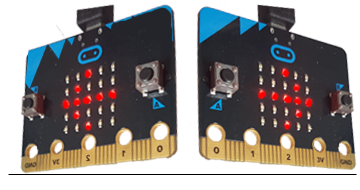Program:

```from microbit import *

while True:
acc = accelerometer.get_x()
print (acc)
if acc > 0:
display.show(Image.ARROW_E)
else:
display.show(Image.ARROW_W)
sleep(100)
```
► Copy to clipboard

Spirit level instrumentHere the micro:bit should work like a spirit level. You use the fact that the x and y components of the accelerations are about 0 when the board is in a horizontal position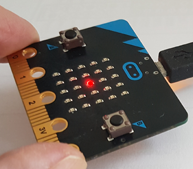Only one pixel is displayed, which shifts to the right, left, up or down depending on the inclination. The aim is to align the spirit level so that the middle LED lights up.

In the program, you use four conditions to determine the x and y values of the pixel, and then clear the pixel before lighting the new one.

Program:

```from microbit import *

x = 1
y = 1

while True:
accX = accelerometer.get_x()
accY = accelerometer.get_y()
if accX > 100 and x < 4:
x += 1
elif accX < -100 and x > 0:
x -= 1
elif accY > 100 and y < 4:
y += 1
elif accY < -100 and y > 0:
y -= 1
display.clear()
display.set_pixel(x, y, 9)
sleep(100)
```
► Copy to clipboard

### MEMO

 With the accelerometer you determine the movements and the position of the micro:bit. Usually you request the sensor value regularly every 10 to 100 milliseconds in a endless loop. You say that you are "polling" sensor values in a measurement loop.

### EXERCISES

1.
 Modify the first example above so that the tilt of the micro:bit in the y-direction is measured and displayed with the arrows Image.ARROW_N, or Image.ARROW_S.

2.
 By tilting the micro:bit, all pixels on the edge should be switched on one by one.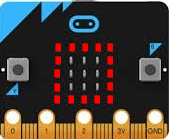3 You want to measure the "shaking" of the micro:bit. To do this, use a = accelerometer.get_values() to get the acceleration components, but only look at the magnitude b of the x and y components, which is calculated as follows b = √ a * a + a * a a) In the terminal window, write out the magnitude in an endless loop 50 times per second. b) After starting the program, execute the measurements for only 1 second and write out the maximum of  b. c) Write the maximum as an integer on the display (as an endless scrolling text). Instructions: To make b an integer, use n = int (b). For the display you have to convert it to a string with s = str(n). d) Create a "shaking game" in which the one who shakes the strongest wins. However to make the game more attractive, the measurement should only start when the character > appears after a random time of 3-6 seconds. It is forbidden to shake before.

3-1
##### Professional advice:

Acceleration generally means the rate of change of velocity, that is, one-dimensionalwhere v is the speed.

3-2
##### Professional advice:

From the three acceleration components you can calculate pitch and roll angles as shown below. Start the following program and tilt the board sideways or forwards and backwards. In the terminal window, the values of pitch and roll are written out in degrees.

```from microbit import *
from math import *

def getPitch():
a = accelerometer.get_values()
pitch = atan2(a, -a)
return int(degrees(pitch))

def getRoll():
a = accelerometer.get_values()
anorm = sqrt(a * a + a * a + a * a)
roll = asin(a / anorm)
return int(degrees(roll))

while True:
pitch = getPitch()
roll = getRoll()
print("p: " + str(pitch))
print("r: " + str(roll))
print()
sleep(100)
```